AP State Board Syllabus AP SSC 10th Class Maths Textbook Solutions Chapter 6 Progressions Ex 6.5 Textbook Questions and Answers.

## AP State Syllabus SSC 10th Class Maths Solutions 6th Lesson Progressions Exercise 6.5

### 10th Class Maths 6th Lesson Progressions Ex 6.5 Textbook Questions and Answers

Question 1.
For each geometric progression find the common ratio ‘r’, and then find an.
i) 3, $$\frac{3}{2}$$, $$\frac{3}{4}$$, $$\frac{3}{8}$$, …….
Given G.P.: 3, $$\frac{3}{2}$$, $$\frac{3}{4}$$, $$\frac{3}{8}$$, …….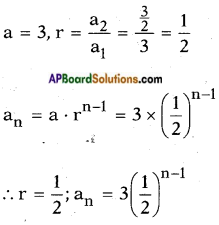ii) 2, -6, 18, -54, …….
Given G.P. = 2, -6, 18, -54, …….
a = 2, r = $$\frac{a_{2}}{a_{1}}$$ = $$\frac{-6}{2}$$ = -3
an = a . rn-1 = 2 × (-3)n-1
∴ r = -3; an = 2(-3)n-1iii) -1, -3, -9, -27, ……
Given G.P. = -1, -3, -9, -27, ……
a = -1, r = $$\frac{a_{2}}{a_{1}}$$ = $$\frac{-3}{-1}$$ = 3
an = a . rn-1 = (-1) × 3n-1
∴ r = 3; an = (-1) × 3n-1

iv) 5, 2, $$\frac{4}{5}$$, $$\frac{8}{25}$$, …….
Given G.P. = 5, 2, $$\frac{4}{5}$$, $$\frac{8}{25}$$, …….
a = 5, r = $$\frac{a_{2}}{a_{1}}$$ = $$\frac{2}{5}$$
an = a . rn-1 = 5 × $$\left(\frac{2}{5}\right)^{n-1}$$
∴ r = $$\frac{2}{5}$$; an = 5$$\left(\frac{2}{5}\right)^{n-1}$$

Question 2.
Find the 10th and nth term of G.P.: 5, 25, 125,…..
Given G.P.: 5, 25, 125,…..
a = 5, r = $$\frac{a_{2}}{a_{1}}$$ = $$\frac{25}{5}$$ = 5
an = a . rn-1 = 5 × (5)n-1 = 51+n-1 = 5n
a10 = a . r9 = 5 × 59 = 510
∴ a10 = 510; an = 5n

Question 3.
Find the indicated term of each geometric progression.
i) a1 = 9; r = $$\frac{1}{3}$$; find a7.
an = a . rn-1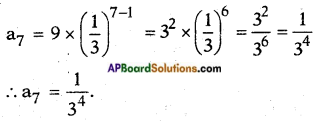ii) a1 = -12; r = $$\frac{1}{3}$$; find a6.
an = a . rn-1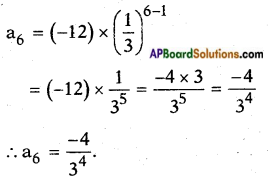Question 4.
Which term of the G.P.
i) 2, 8, 32,….. is 512?
Given G.P.: 2, 8, 32,….. is 512
a = 2, r = $$\frac{a_{2}}{a_{1}}$$ = $$\frac{8}{2}$$ = 4
Let the nth term of G.P. be 512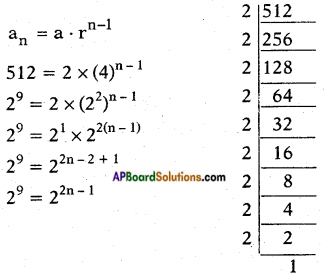512 = 2 × 2 × 2 × 2 × 2 × 2 × 2 × 2 × 2
= 29
∴ 2n – 1 = 9
[∵ bases are equal, exponents are also equal]
∴ 2n = 9 + 1 = 10
n = $$\frac{10}{2}$$ = 5
∴ 512 is the 5th term of the given G.P.

ii) √3, 3, 3√3, …….. is 729?
Given G.P.: √3, 3, 3√3, …….. is 729
a = √3, r = $$\frac{a_{2}}{a_{1}}$$ = $$\frac{3}{\sqrt{3}}$$ = √3
now an = a . rn-1 = 729
⇒ (√3)(√3)n-1 = 729
⇒ (√3)n = 36 = (√3)12
⇒ n = 12
So 12th term of GP √3, 3, 3√3, …….. is 729.

iii) $$\frac{1}{3}$$, $$\frac{1}{9}$$, $$\frac{1}{27}$$, ……. is $$\frac{1}{2187}$$?
Given G.P.: $$\frac{1}{3}$$, $$\frac{1}{9}$$, $$\frac{1}{27}$$, ……. is $$\frac{1}{2187}$$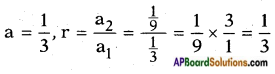Let $$\frac{1}{2187}$$ be the nth term of the G.P., then
an = a . rn-1 = $$\frac{1}{2187}$$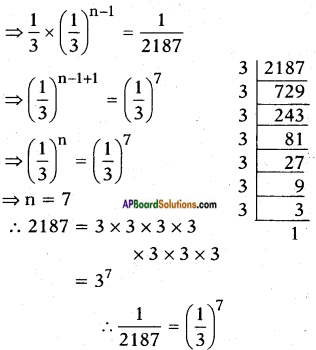[∵ bases are equal, exponents are also equal]
7th term of G.P is $$\frac{1}{2187}$$.Question 5.
Find the 12th term of a G.P. whose 8 term is 192 and the common ratio is 2.
Given a G.P. such that a8 = 192 and r = 2
an = a . rn-1
a8 = a . (2)8-1 = 192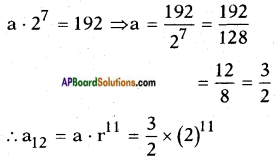= 3 × 210 = 3 × 1024 = 3072.

Question 6.
The 4th term of a geometric progression is $$\frac{2}{3}$$ and the seventh term is $$\frac{16}{81}$$. Find the geometric series.
Given: In a G.P.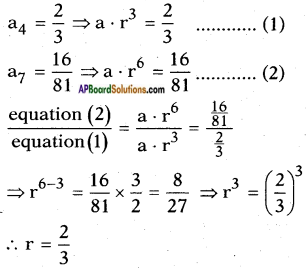Now substituting r = $$\frac{2}{3}$$ in equation (1)
we get,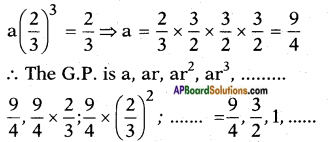Question 7.
If the geometric progressions 162, 54, 18, ….. and $$\frac{2}{81}$$, $$\frac{2}{27}$$, $$\frac{2}{9}$$,….. have their nth term equal, find the value of n.
Given G.P.: 162, 54, 18, ….. and $$\frac{2}{81}$$, $$\frac{2}{27}$$, $$\frac{2}{9}$$,……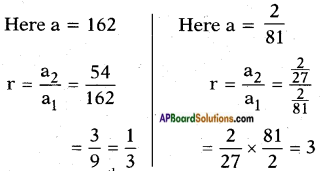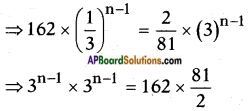⇒ n = $$\frac{10}{2}$$ = 5